# How Block Statistics works

The Block Statistics tool performs a neighborhood operation that calculates a statistic for input cells within a fixed set of non-overlapping windows or neighborhoods. The statistic (for example, maximum, average, or sum) is calculated for all input cells contained within each neighborhood. The resulting value for an individual neighborhood or block is assigned to all cell locations contained in the minimum bounding rectangle of the specified neighborhood.

Since the neighborhoods do not overlap, any particular cell will be included in the calculations for one block only.

The shape of a neighborhood can be an annulus (a donut), a circle, a rectangle, or a wedge. The possible statistics that can be calculated within a neighborhood are mean, majority, maximum, median, minimum, minority, range, standard deviation, sum, and variety.

Conceptually, the Block Statistics tool works as follows:

• It creates the first specified neighborhood—for example, a circular neighborhood—in the top left corner of the analysis window.

• It calculates the minimum bounding rectangle to determine the size of the output block.

• It partitions the remaining area of the raster into defined blocks. Blocks cannot overlap.

• It identifies in each block the cell locations that will be used in the block calculations. The cell locations are determined by the definition of the specified neighborhood—for example, a circular neighborhood—that fits into the bounding rectangle.

• It calculates the output value for each neighborhood of each block. The resultant values are assigned to every cell location in the corresponding output block.

## Neighborhood types

The shape of a neighborhood can be an annulus (a donut), a circle, a rectangle, or a wedge. Using a kernel file, you can also define a custom neighborhood shape, as well as assign different weights to specific cells in the neighborhood before the statistic is calculated.

Following are descriptions of the neighborhood shapes and how they are defined:

• Annulus
• The annulus shape is composed of two circles, one inside the other to make a donut shape. Cells with centers that fall outside the radius of the smaller circle but inside the radius of the larger circle will be included in processing the neighborhood. Therefore, the area that falls between the two circles constitutes the annulus neighborhood.
• The radius is identified in cells or map units, measured perpendicular to the x- or y-axis. When the radii are specified in map units, they are converted to radii in cell units. The resulting radii in cell units produce an area that most closely represents the area calculated using the original radii in map units. Any cell center encompassed by the annulus will be included in the processing of the neighborhood.
• The default annulus neighborhood is an inner radius of one cell and an outer radius of three cells.
• An example illustration of an annulus neighborhood follows: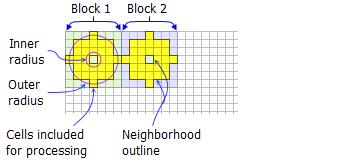Yellow shading indicates the cells that will be included in the calculations for an example annulus block neighborhood (inner radius = 1 cell, outer radius = 3 cells).

• Circle
• A circle neighborhood is created by specifying a radius value.
• The radius is identified in cell or map units, measured perpendicular to the x- or y-axis. When the radius is specified in map units, additional logic is used to determine which cells are included in the processing neighborhood. First, the exact area of a circle defined by the specified radius value is calculated. Next, the area is calculated for two additional circles, one with the specified radius value rounded down and one with the specified radius value rounded up. These two areas are compared to the result from the specified radius, and the radius of the area that is closest will be used in the operation.
• The default circle neighborhood radius is three cells.
• An example illustration of a circle neighborhood follows: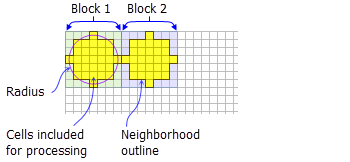Yellow shading indicates the cells that will be included in the calculations for an example circle block neighborhood (radius = 3 cells).

• Rectangle
• The rectangle neighborhood is specified by providing a width and a height in either cells or map units.
• Only the cells with centers that fall within the defined object are processed as part of the rectangle neighborhood.
• The default rectangle neighborhood is a square with a height and width of three cells.
• An example illustration of a rectangle neighborhood follows: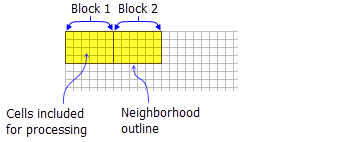Yellow shading indicates the cells that will be included in the calculations for an example rectangle block neighborhood (width = 6 cells, height = 4 cells).

• Wedge
• A wedge is a pie-shaped neighborhood specified by a radius, a starting angle, and an ending angle.
• The wedge extends counterclockwise from the starting angle to the ending angle. Angles are specified in arithmetic degrees from 0 to 360, where 0 is on the positive x-axis (3:00 on a clock), and can be integer or floating point. Negative angles can be used.
• The radius is identified in cells or map units, measured perpendicular to the x- or y-axis. When the radius is specified in map units, it is converted to a radius in cell units. The resulting radius in cell units produces an area that most closely represents the area calculated using the original radius in map units. Any cell center encompassed by the wedge will be included in the processing of the neighborhood.
• The default wedge neighborhood is from 0 to 90 degrees, with a radius of three cells.
• An example illustration of a wedge neighborhood follows: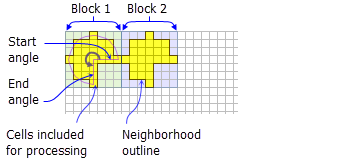Yellow shading indicates the cells that will be included in the calculations for an example wedge block neighborhood (radius = 3 cells, start angle = 0, end angle = 270).

• Irregular
• Allows you to specify an irregularly shaped neighborhood.
• The irregular kernel file specifies which cell positions should be included within the neighborhood.
• For the kernel file for an irregular neighborhood:

• The irregular kernel file is an ASCII text file that defines the values and shape of an irregular neighborhood. The file can be created with any text editor.
• The first line specifies the width and height of the neighborhood (the number of cells in the x direction, followed by a space, and the number of cells in the y direction).
• The subsequent lines give the values of each position in the neighborhood. The values are input in the same configuration as appears in the neighborhood they represent. A space between each value is necessary.
• The values in the kernel file should be either 0 (zero) or 1 (one). However, any value not equal to 0 will be interpreted as 1.
• A value of 0 (not a blank space) for a cell position indicates that the cell is not a member of the neighborhood and will not be used for processing. A value of 1 indicates that its corresponding cell (and value) is a member of the neighborhood.

• An example of an ASCII irregular kernel file and the neighborhood it represents follows: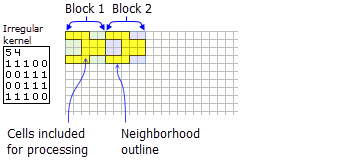Yellow shading indicates the cells that will be included in the calculations for an example irregular block neighborhood.

• Weight
• Similar to the irregular neighborhood type, the weight neighborhood allows you to define an irregular neighborhood, but additionally allows you to apply weights to the input values.
• The weight kernel file specifies which cell positions should be included within the neighborhood and the weights by which they will be multiplied.
• The weight neighborhood is only available for the mean, standard deviation, and sum statistics types.
• For the kernel file for a weighted neighborhood:

• The weight kernel file is an ASCII text file that defines the values and shape of a weight neighborhood. The file can be created with any text editor.
• The first line specifies the width and height of the neighborhood (the number of cells in the x direction, followed by a space, and the number of cells in the y direction).
• The subsequent lines give the weight values of each position in the neighborhood. The values are input in the same configuration as appears in the neighborhood they represent. Positive, negative, and decimal values are all valid options to use as a weight. A space between each value is necessary.
• For locations in the neighborhood that are not to be part of the calculation, use a value of 0 at the corresponding location in the kernel file.

• An example of an ASCII-weighted kernel file and the neighborhood it represents follows: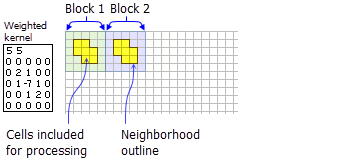Yellow shading indicates the cells that will be included in the calculations for an example weighted block neighborhood.

## Statistics type

The available statistics are majority, maximum, mean, median, minimum, minority, range, standard deviation, and sum. The default statistics type is mean.

• Majority
• Only an integer raster can be used as input.
• For this statistic, the frequency of each unique cell value in each block neighborhood is determined first. If there is a single value that has the highest frequency (is the most common), that value is returned as the output for each cell of that block. However, there can be a tie, where there are two or more input values that have the highest frequency. In this case, all of the output cells in that block will receive NoData.
• Maximum
• If the input raster is integer, the values on the output raster will be integer; if the values on the input are floating point, the values on the output will be floating point.
• Mean
• The output raster will always be floating point.
• The mean statistic can be used with the weight neighborhood type.
• Median
• Only an integer raster can be used as input.
• When the number of valid cell values in the neighborhood is odd, the median value is calculated by ranking the values and selecting the middle value. If the number of values in a neighborhood is even, the values will be ranked, and the middle two values will be averaged and rounded down to the nearest integer.
• Minimum
• If the input raster is integer, the values on the output raster will be integer; if the values on the input are floating point, the values on the output will be floating point.
• Minority
• Only an integer raster can be used as input.
• For this statistic, the frequency of each unique cell value in each block neighborhood is first determined. If there is a single value that has the lowest frequency (is the least common), that value is returned as the output for each cell of that block. However, there can be a tie, where there are two or more input values that have the same lowest frequency. In this case, all of the output cells in that block will receive NoData.
• Range
• If the input raster is integer, the values on the output raster will be integer; if the values on the input are floating point, the values on the output will be floating point.
• The values for each cell location on the output raster are determined on a cell-by-cell basis by applying this simple formula: Block Range = Block Maximum – Block Minimum.
• Standard deviation
• The output raster will always be floating point.
• The Standard deviation statistic can be used with the weight neighborhood type.
• Note that the standard deviation is calculated on the entire population (the N method); it is not estimated based on a sample (the N-1 method).
• Sum
• If the input raster is integer, the values on the output raster will be integer; if the values on the input are floating point, the values on the output will be floating point.
• Variety
• Only an integer raster can be used as input.

## Processing cells of NoData

The Ignore NoData in calculations option controls how NoData cells within the neighborhood window are handled. When this option is checked (the DATA option), any cells in the neighborhood that are NoData will be ignored in the calculation of the output cell value. When unchecked (the NODATA option), if any cell in the neighborhood is NoData, the output cell will be NoData.

## Uses for block statistics

The Block Statistics tool can be used instead of the Resample tool to resample a raster from a fine resolution to a coarser one. Instead of using the nearest neighbor, bilinear, or cubic resampling techniques, it may be preferable to assign the coarser raster cells the maximum, minimum, or average of the values in the new geographic extent that the coarser cells encompass. To do so, the appropriate statistics are applied to the block—the average (mean) or maximum, for example.

The Aggregate tool from the Generalization toolset is similar to Block Statistics in that it allows for the aggregation of cell locations based on the sum, mean, median, or minimum or maximum values within a spatial window, which is determined by the desired output resolution. There are two major differences between the two options, however:

• The output raster resulting from the Aggregate tool is resampled to the desired resolution.
• There is no concept of a specified neighborhood in the Aggregate tool. The neighborhood and the output block are the same, are always rectangular, and encompass the same cell locations. The size of the block in the Aggregate tool is determined by the aggregation of cells necessary to reach the desired resolution.